# Derivative (mathematics) facts for kids

Kids Encyclopedia Facts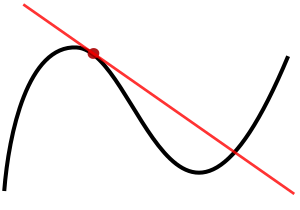A function (black) and a tangent (red). The derivative at the point is the slope of the tangent.

In mathematics (particularly in differential calculus), the derivative is a way to show instantaneous rate of change: that is, the amount by which a function is changing at one given point. For functions that act on the real numbers, it is the slope of the tangent line at a point on a graph. The derivative is often written as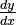$\tfrac{dy}{dx}$ ("dy over dx", meaning the difference in y divided by the difference in x). The d is not a variable, and therefore cannot be cancelled out. Another common notation is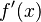$f'(x)$—the derivative of function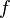$f$ at point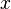$x$.

## Definition of a derivative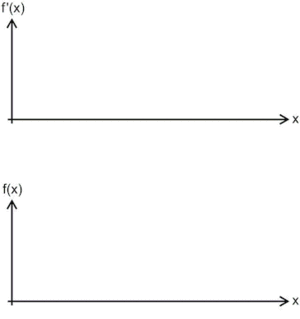An animation giving an intuitive idea of the derivative, as the "swing" of a function change when the argument changes.

The derivative of y with respect to x is defined as the change in y over the change in x, as the distance between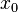$x_0$ and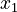$x_1$ becomes infinitely small (infinitesimal). In mathematical terms,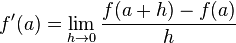$f'(a)=\lim_{h\to 0}{\frac{f(a+h)-f(a)}{h}}$

That is, as the distance between the two x points (h) becomes closer to zero, the slope of the line between them comes closer to resembling a tangent line.

## Derivatives of functions

### Linear functions

Derivatives of linear functions (functions of the form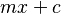$mx+c$ with no quadratic or higher terms) are constant. That is, the derivative in one spot on the graph will remain the same on another.

When the dependent variable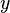$y$ directly takes$x$'s value (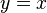$y=x$), the slope of the line is 1 in all places, so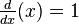$\tfrac{d}{dx}(x) = 1$ regardless of where the position is.

When$y$ modifies$x$'s number by adding or subtracting a constant value, the slope is still 1, because the change in$x$ and$y$ do not change if the graph is shifted up or down. That is, the slope is still 1 throughout the entire graph and its derivative is also 1.

### Power functions

Power functions (in the form of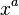$x^a$) behave differently from linear functions, because their exponent and slope vary.

Power functions, in general, follow the rule that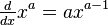$\tfrac{d}{dx}x^a = ax^{a-1}$. That is, if we give a the number 6, then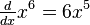$\tfrac{d}{dx} x^6 = 6x^5$

Another example, which is less obvious, is the function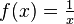$f(x) = \tfrac{1}{x}$. This is essentially the same, because 1/x can be simplified to use exponents: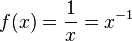$f(x) = \frac{1}{x} = x^{-1}$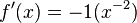$f'(x) = -1(x^{-2})$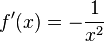$f'(x) = -\frac{1}{x^2}$

In addition, roots can be changed to use fractional exponents, where their derivative can be found: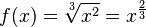$f(x) = \sqrt{x^2} = x^\frac{2}{3}$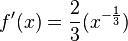$f'(x) = \frac{2}{3}(x^{-\frac{1}{3}})$

### Exponential functions

An exponential is of the form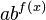$ab^{f\left(x\right)}$, where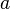$a$ and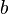$b$ are constants and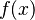$f(x)$ is a function of$x$. The difference between an exponential and a polynomial is that in a polynomial$x$ is raised to some power, whereas in an exponential$x$ is in the power.

#### Example 1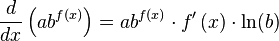$\frac{d}{dx}\left( ab^{ f\left( x \right) } \right) = ab^{f(x)} \cdot f'\left(x \right) \cdot \ln(b)$

#### Example 2

Find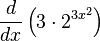$\frac{d}{dx} \left( 3\cdot2^{3{x^2}} \right)$.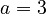$a = 3$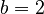$b = 2$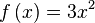$f\left( x \right) = 3x^2$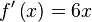$f'\left( x \right) = 6x$

Therefore,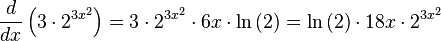$\frac{d}{dx} \left(3 \cdot 2^{3x^2} \right) = 3 \cdot 2^{3x^2} \cdot 6x \cdot \ln \left( 2 \right) = \ln \left(2 \right) \cdot 18x \cdot 2^{3x^2}$

### Logarithmic functions

The derivative of logarithms is the reciprocal: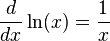$\frac{d}{dx}\ln(x) = \frac{1}{x}$.

Take, for example,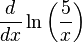$\frac{d}{dx}\ln\left(\frac{5}{x}\right)$. This can be reduced to (by the properties of logarithms):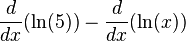$\frac{d}{dx}(\ln(5)) - \frac{d}{dx}(\ln(x))$

The logarithm of 5 is a constant, so its derivative is 0. The derivative of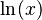$\ln(x)$ is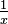$\tfrac{1}{x}$. So,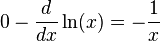$0 - \frac{d}{dx} \ln(x) = -\frac{1}{x}$

For derivatives of logarithms not in base e, such as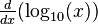$\tfrac{d}{dx}(\log_{10}(x))$, this can be reduced to: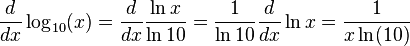$\frac{d}{dx} \log_{10}(x) = \frac{d}{dx} \frac{\ln{x}}{\ln{10}} = \frac{1}{\ln{10}} \frac{d}{dx} \ln{x} = \frac{1}{x \ln(10)}$

### Trigonometric functions

The cosine function is the derivative of the sine function, while the derivative of cosine is negative sine (provided that x is measured in radians):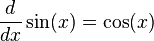$\frac{d}{dx}\sin(x) = \cos(x)$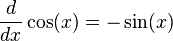$\frac{d}{dx}\cos(x) = -\sin(x)$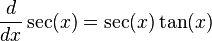$\frac{d}{dx}\sec(x) = \sec(x)\tan(x)$.

## Properties of derivatives

Derivatives can be broken up into smaller parts where they are manageable (as they have only one of the above function characteristics). For example,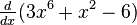$\tfrac{d}{dx}(3x^6 + x^2 - 6)$ can be broken up as: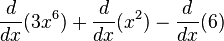$\frac{d}{dx}(3x^6) + \frac{d}{dx}(x^2) - \frac{d}{dx}(6)$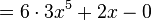$= 6 \cdot 3x^5 + 2x - 0$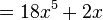$= 18x^5 + 2x\,$

## Uses of derivatives

A function's derivative can be used to search for the maxima and minima of the function, by searching for places where its slope is zero.

Derivatives are used in Newton's method, which helps one find the zeros (roots) of a function..One can also use derivatives to determine the concavity of a function, and whether the function is increasing or decreasing.

## Images for kidsDerivative (mathematics) Facts for Kids. Kiddle Encyclopedia.